﻿ 位移法测量透镜焦距的最佳位置分析 Analysis of Optimal Position for Measuring Focal Length of Convex Lens by Displacement Method

Applied Physics
Vol. 09  No. 04 ( 2019 ), Article ID: 29676 , 6 pages
10.12677/APP.2019.94019

Analysis of Optimal Position for Measuring Focal Length of Convex Lens by Displacement Method

Ye Liu, Cunhai Liu, Kun Wang

Aeronautical Basic College, Naval Aviation University, Yantai Shandong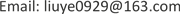Received: Mar. 22nd, 2019; accepted: Apr. 5th, 2019; published: Apr. 12th, 2019ABSTRACT

In this paper, the focal length of thin convex lens is measured by displacement method and the calculation method of focal length error is discussed. The measurement error caused by position judgment of clear image is discussed, and the influence of object distance on focal length measurement error of the lens is analyzed. The accurate range of focal length determination of thin convex lens is obtained.

Keywords:Convex Lens, Focal Length, Displacement Method, Error AnalysisCopyright © 2019 by author(s) and Hans Publishers Inc.1. 引言

2. 位移法测量薄透镜焦距

2.1. 基本原理

$f=\frac{{L}^{2}-{d}^{2}}{4L}$ (1)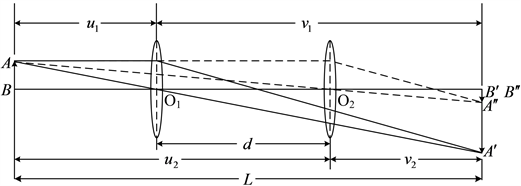Figure 1. Measuring principle diagram of convex lens by displacement method

2.2. 测量方法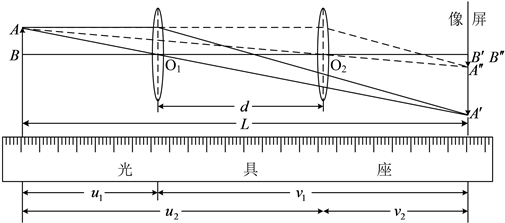Figure 2. Experimental device for focal length of convex lens measured by displacement method

$\frac{1}{{u}_{1}}+\frac{1}{{v}_{1}}=\frac{1}{f}$ (2)(3)

${u}_{1}+{u}_{2}=L$ ${u}_{2}-{u}_{1}=d$

${v}_{1}+{v}_{2}=L$ ${v}_{2}-{v}_{1}=d$

2.3. 测量数据及数据处理

Table 1. List of data for lens focal length measurement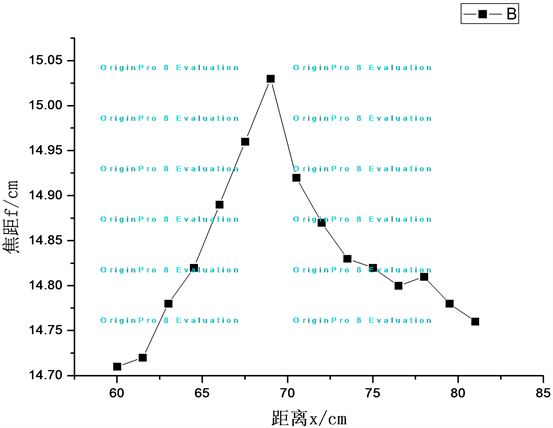Figure 3. Relationship between lens focal length and object and screen distanceTable 2. List of relative error and material distance data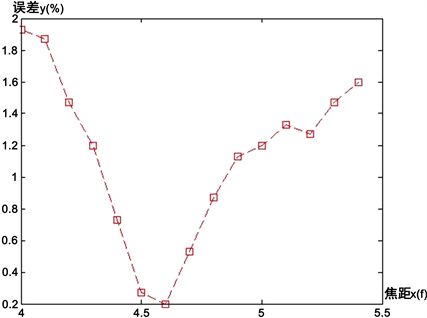Figure 4. Relation between relative error and object and screen distance

3. 实验结果分析

4. 结论

Analysis of Optimal Position for Measuring Focal Length of Convex Lens by Displacement Method[J]. 应用物理, 2019, 09(04): 157-162. https://doi.org/10.12677/APP.2019.94019

1. 1. 苏大图. 光学测试技术[M]. 北京: 北京理工大学出版社, 1996: 116-122.

2. 2. 何俊芳, 朱海永, 等. 激光热透镜焦距测量实验及其公式改进[J]. 物理实验, 2011, 31(11): 22-24.

3. 3. 岳巾英, 刘华, 许文斌, 等. 计算全息法测量长焦透镜面形和焦距[J]. 中国光学与应用光学, 2009, 2(6): 502-507.

4. 4. 王云创, 李得尧, 邢娟. 光心指示偏差与焦距测量误差关系的研究[J]. 物理通报, 2001(3): 24-25.

5. 5. 王云创, 刘俊杰, 等. 透镜倾斜对焦距测量误差的影响[J]. 物理与工程, 2016, 26(3): 45-48.

6. 6. 王云创. 几何光学实验中一现象的理论描述和验证[J]. 物理通报, 2002(5): 23-24.

7. 7. 顾菊观, 钱淑珍. 凸透镜焦距测量方法的探索[J]. 大学物理实验, 2014(1): 49-51.

8. 8. 李伟, 张丽巍, 等. 薄透镜焦距测量方法的研究[J]. 大学物理实验, 2014, 27(1): 34-36.

9. 9. 李伟, 刘超, 等. 薄透镜焦距测量方法的研究[J]. 物理实验, 2014, 34(7): 27-29.

10. 10. 翁存程, 林莉玲. 薄透镜成像实验中的共轴调节[J]. 大学物理实验, 2010, 23(4): 40-42.

11. 11. 葛国芳. “透镜焦距测量”实验中物屏的改进[J]. 大学物理实验, 2011, 24(4): 29-30.